Courses

# Spillways and Energy Dissipators (Part - 7) Civil Engineering (CE) Notes | EduRev

## Civil Engineering (CE) : Spillways and Energy Dissipators (Part - 7) Civil Engineering (CE) Notes | EduRev

The document Spillways and Energy Dissipators (Part - 7) Civil Engineering (CE) Notes | EduRev is a part of Civil Engineering (CE) category.
All you need of Civil Engineering (CE) at this link: Civil Engineering (CE)

Design of Hydraulic Jump Stilling Basin type energy dissipators

A hydraulic jump is the sudden turbulent transition of supercritical flow to subcritical. This phenomena, which involves a loss of energy, is utilized at the bottom of a spillway as an energy dissipator by providing a floor for the hydraulic jump to take place (Figure 47). The amount of energy dissipated in a jump increases with the rise in Froude number of the supercritical flow.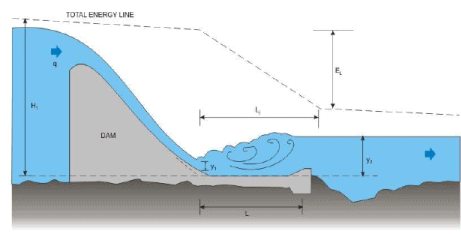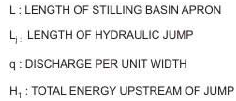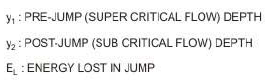Figure 47. Definition sketch of hydraulic jump & associated parameters

The two depths, one before (y1and one after (y2the jump are related by the following expression: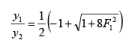(14)

Where F1 is the incoming Froude number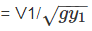Alternatively, the expression may be written in terms of the outgoing Froude number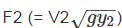as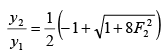(15)

where V1 and Vare the incoming and outgoing velocities and g is the acceleration due to gravity.

The energy lost in the hydraulic jump (EL) is given as: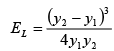(16)

In most cases, it is possible to find out the pre-jump depth (y1) and velocity (V1) from the given value of discharge per unit width (q) through the spillway. This is done by assuming the total energy is nearly constant right from the spillway entrance up to the beginning of the jump formation, as shown in Figure 47. V1 may be assumed to be equal to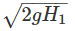, where H1 is the total energy upstream of the spillway, and neglecting friction losses in the spillway. The appropriate expressions may be solved to find out the postjump depth (y2) and velocity (V2).

The length of the jump (Lj) is an important parameter affecting the size of a stilling basin in which the jump is used. There have been many definitions of the length of the jump, but it is usual to take the length to be the horizontal distance between the toe of the jump upto a section where the water surface becomes quite level after reaching a maximum level. Because the water surface profile is very flat towards the end of the jump, large personal errors are introduced in the determination of the jump length.

Bradley and Peterka (1975) have experimentally found the length of hydraulic jumps and plotted them in terms of the incoming Froude number (F1), and post-jump depth (y2) as shown in Figure 48. It is evident that while Lj/y2 varies most for small values of F1, at higher values, say above 5 or so, Lj /y2 is practically constant at a value of about 6.1.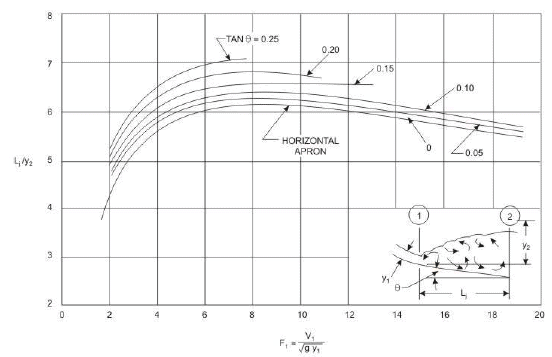Figure 48. Length of hydraulic jump on a horizontal or inclined floor

The depth of water in the actual river downstream of the stilling basin (y2*) is determined from the river flow observations that have been plotted as a stage-discharge curve (Figure 49).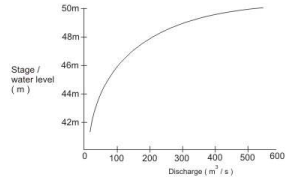Subtracting the stilling basing apron level from the stage or water level corresponding to the total discharge passing through the spillway gives the tail-water depth (y2*). Since the stage-discharge curve gives indications about the tail-water of the spillway, it is called the Tail-Water Rating Curve (TRC), usually expressed as the water depth (y2*) versus unit discharge (q), as shown in Figure 50(a).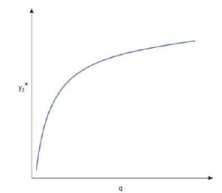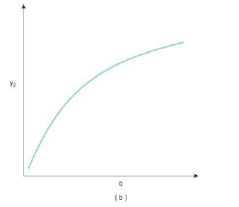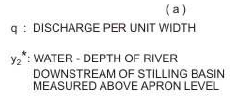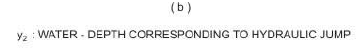Figure 50. Water Level curves ( a ): Tail - water Rating Curve (TRC) ( b ): Jump Rating Curve (JRC)

At the same time, using the formula relating unit discharge (q) with the post-jump depth (y2), a similar graph may be obtained, as shown in Figure 50(b). Since this graph gives indication about the variation of the post-jump depth, it is called the Jump Rating Curve (JRC).

In general, the JRC and TRC would rarely coincide, if plotted on the same graph, as shown in Figure 51.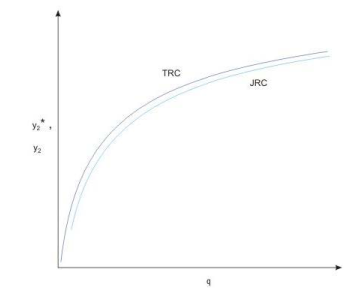Figure 51. TRC & JRC coinciding

At times, the TRC may lie completely below the JRC (Figure 52), for all discharges, in which case the jump will be located away from the toe of the spillway resulting in possible erosion of the riverbed.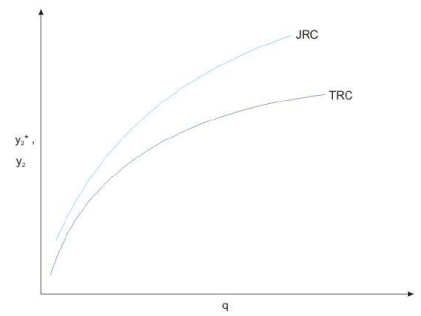Figure 52. TRC below JRC for all discharges

If the TRC is completely above the jump would be located so close to the spillway to make it submerged  which may not dissipate the energy completely. (Figure 53)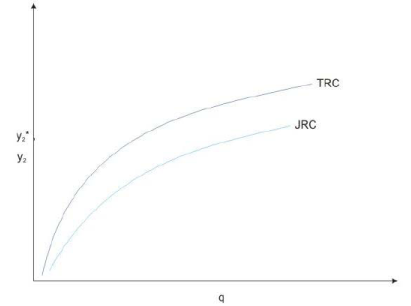Figure 53. TRC above JRC for all discharges

It may also be possible in actual situations that the TRC may be below the JRC for some discharges above for the rest, as shown in Figs. 54 and 55.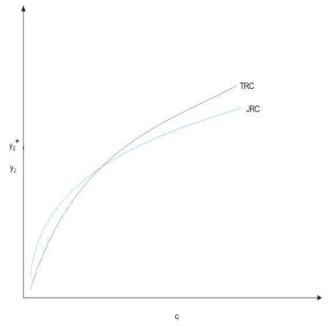Figure 54. TRC below JRC for low discharges and above for high discharges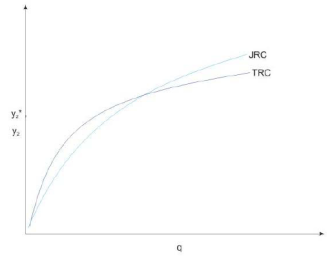Figure 55. TRC above JRC for low discharges and below for high discharges

In these cases two, favourable location of jump may not be possible. In view of the above situations, the following recommendations have been made for satisfactory performance of the hydraulic jumps.

Case1 (Figure 51) This is the ideal case in which the horizontal apron provided on the riverbed downstream from the toe of the spillway would suffice. The length of the apron should be equal to the length of the jump corresponding to the maximum discharge over the spillway.

Case2 (Figure 52) It is apparent that the tail water depth as provided by the natural river is not sufficiently for the jump to form. This may be over come by providing a stilling basin apron that is depressed below the average riverbed level (Figure56) or by providing a sill or baffle of sufficient height at the end of the spillway (Figure 57)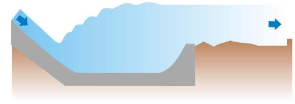Figure 56. Depressed floor of stilling basin apron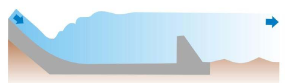Figure 57. High end - sill or baffle at toe of stilling basin

Case 3 (Figure 53) Since this situation results in submergence results in submergence of the jump, it is necessary to raise the floor in order to form a clear jump. In practice, it is done by providing an inclined apron of the stilling basin (Figure 58).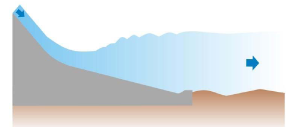Figure 58. Inclined stilling basin

Case 4 (Figure 54) This situation may be taken care of by providing an inclined floor in the upper portion of the stilling basin and providing either a depressed floor in the lower portion of the basin or provide a baffle at the end of the basin.

Case 5 (Figure 55) In this case a sloping apron may be provided which lies partly above and partly below the riverbed. So that the jump will form on the higher slope at low discharges and on the lower slope at high discharges. The type of Stilling Basins that may be provided under different situations is recommended by the Bureau of Indian Standards code IS: 4997-1968 “Criteria for design of hydraulic jump type stilling basins with horizontal and sloping aprons”. In all, these are four types of basin shapes recommended. Types I and II are meant for basins with horizontal floors and types III and IV for basins with inclined floors.

Offer running on EduRev: Apply code STAYHOME200 to get INR 200 off on our premium plan EduRev Infinity!

,

,

,

,

,

,

,

,

,

,

,

,

,

,

,

,

,

,

,

,

,

;